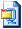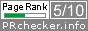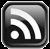# LEARN MS-ACCESS TIPS AND TRICKS

Learn Microsoft Access Advanced Programming Techniques, Tips and Tricks.

### Introduction.

This is the continuation of a series of different 3D-Heading-Styles introduced for designing Microsoft Access Form/Report Headings. This design is a variant of the 3D-Heading Style presented under the Title Create 3D Headings on Forms. Both the styles have their own beauty and once they are created you may copy the same controls and customize them with different fore-color, font & font styles (Bold, Italics, etc.) and use it on form or Report Headings.

I have several of this type of designs and if this is the first one you came across on this site then you must prepare your MS-Access Project by adding few Library Files and the main programs of this series (if you have not already done) before you are able to run the Code for this Heading Style and others presented on this website. Follow the steps given below:

1. Link a few Common Library Files (they are already there in your system, you only need to attach them) to your Project by following the steps described in my earlier post with the Title Command-Button Animation
2. ### The VBA Programs

3. Copy the following main program Codes given below into a new Global VBA Module of your Project and save it.

If you have already copied them from earlier Posts then copy only the last Function: Shadow3D()

```Option Compare Database
Option Explicit
'-- Global declarations
Private Const lngheight as long = 0.45 * 1440
Private Const lngWidth as long = 4.5 * 1440
Private Const intFontSize as integer = 26
Private Const intTextAlign as integer = 0
Private Const intBackStyle as integer = 0
Private Const LngI as long = 0.0104 * 1440
Private Const intX as long = 0.15 * 1440
Private Const intY as long = 0.15 * 1440
Dim MyFrm As Form

Public Function FormTxtLabels(Optional ByVal ControlType As Integer) As String
'---------------------------------------------------
'Author : a.p.r. pillai
'Date : September 2006
'ControlType = 0 for label
'ControlTYPE = 1 for TextBox
'---------------------------------------------------
Dim ctl As Control
'On Error GoTo FormTxtLabels_Err
If ControlType > 0 Then ControlType = 1

Set MyFrm = CreateForm

If ControlType = 1 Then
Set ctl = CreateControl(MyFrm.NAME, acTextBox, acDetail, , , (0.2 * 1440), (0.2 * 1440), lngWidth, lngheight)

With ctl
.ControlSource = "=" & Chr(34) & "msaccesstips.com" & Chr(34)
End With
Else
Set ctl = CreateControl(MyFrm.NAME, acLabel, _acDetail, , , (0.2 * 1440), (0.2 * 1440), lngWidth, lngheight)

With ctl
.Caption = "msaccesstips.com"
End With

End If

FormTxtLabels = MyFrm.NAME

FormTxtLabels_Exit:
Exit Function
```

```Public Function Validate_Dup(ByRef MyFrm As Form, ByVal intNooflabels As Integer) As Integer
'---------------------------------------------------
'Author : a.p.r. pillai
'Date : September 2006
'ControlType = 0 for label
'ControlTYPE = 1 for TextBox
'---------------------------------------------------
Dim mysec As Section, lblcount As Integer
Dim myctrl As Control, newctrl As Control, j As Integer
Dim lngx As Long, lngY As Long, lngH As Long, lngW As Long
Dim strCap As String, ctrltype As Integer, intlbls As Integer
Dim ctrlName() As String, ctrlIndex() As Integer, i As Long
Dim strFont As String, intFntSize As Integer, x As Integer
Dim intFntWeight As Integer

'On Error GoTo Validate_Dup_Err

Set mysec = MyFrm.Section(acDetail)
intlbls = mysec.Controls.Count - 1

Set myctrl = mysec.Controls(0)
ctrltype = myctrl.ControlType
intNooflabels = intNooflabels - 1
If intlbls > 0 Then
ReDim ctrlName(intlbls) As String
ReDim ctrlIndex(intlbls) As Integer
End If
If ctrltype = 109 And intlbls > 0 Then
For j = 0 To intlbls
Set myctrl = mysec.Controls(j)
ctrlIndex(j) = myctrl.ControlType
ctrlName(j) = myctrl.NAME
Next
For j = 0 To intlbls
If ctrlIndex(j) = 100 Then
DeleteControl MyFrm.NAME, ctrlName(j)
End If
Next
intlbls = mysec.Controls.Count - 1
End If

Set myctrl = mysec.Controls(0)
If intlbls < intNooflabels Then
With myctrl
lngx = .Left
lngY = .Top
lngW = .Width
lngH = 0.0208 * 1440 ' 0.0208 inches
strFont = .FontName
intFntSize = .FontSize
intFntWeight = .FontWeight
End With

If ctrltype = 100 Then
strCap = myctrl.Caption
ElseIf ctrltype = 109 Then
strCap = myctrl.ControlSource
End If
If ctrltype = 109 And intlbls > 0 Then
For j = 0 To intlbls
Set myctrl = mysec.Controls(j)
ctrlIndex(j) = myctrl.ControlType
ctrlName(j) = myctrl.NAME
Next
For j = 0 To intlbls
If ctrlIndex(j) = 100 Then
DeleteControl MyFrm.NAME, ctrlName(j)
End If
Next
intlbls = mysec.Controls.Count - 1
Set myctrl = mysec.Controls(intlbls)
With myctrl
lngx = .Left
lngY = .Top
lngW = .Width
lngH = .Height
ctrltype = .ControlType
strFont = .FontName
intFntSize = .FontSize
intFntWeight = .FontWeight
End With
End If

i = 0.0104 * 1440 + lngH ' 0.0104 inches
lngY = lngY + i
For j = intlbls + 1 To intNooflabels

Set newctrl = CreateControl(MyFrm.NAME, ctrltype, _acDetail, "","", lngx, lngY, lngW, lngH)

If ctrltype = 100 Then
newctrl.Caption = strCap
newctrl.FontName = strFont
newctrl.FontSize = intFntSize
newctrl.FontWeight = intFntWeight
Else
newctrl.ControlSource = strCap
newctrl.FontName = strFont
newctrl.FontSize = intFntSize
newctrl.FontWeight = intFntWeight
End If
lngY = lngY + i
Next
End If

If intlbls > intNooflabels Then
For j = intNooflabels + 1 To intlbls
Set myctrl = mysec.Controls(j)
ctrlIndex(j) = myctrl.ControlType
ctrlName(j) = myctrl.NAME
Next
For j = intNooflabels + 1 To intlbls
DeleteControl MyFrm.NAME, ctrlName(j)
Next
Validate_Dup = 0
End If

intlbls = mysec.Controls.Count - 1
Set myctrl = mysec.Controls(0)
ctrltype = myctrl.ControlType
If ctrltype = 109 Then
For j = 0 To intlbls
Set myctrl = mysec.Controls(j)
With myctrl
.Enabled = False
.Locked = True
.SpecialEffect = 0
End With
Next
End If
Validate_Dup = 0

Validate_Dup_Exit:
Exit Function

Validate_Dup_Err:
MsgBox Err.Description, ,"Validate_Dup"
Validate_Dup = 1
Resume Validate_Dup_Exit
End Function
```

```Public Function MsgLabel()
'------------------------------------------------------------
'Author : a.p.r. pillai
'Date : September 2006
'------------------------------------------------------------
Dim mySection As Section, ctl As Control, xForm As Form
Dim l As Long, t As Long, w As Long, h As Long, F As Long
F = 1440
l = 0.5 * F: t = 1.2 * F: w = 3.6563 * F: h = 0.4896 * F 'values in inches
On Error Resume Next
Set mySection = MyFrm.Section(acDetail)
Set ctl = CreateControl(MyFrm.NAME, acLabel, _acDetail, , "", l, t, w, h)
ctl.Caption = "Click outside the Controls and Drag Over. "  & "Display the Property Sheet. " & "Type New Text for Caption/Control " & "Source Property area for Label/ Text Boxes. " & "Copy and Paste the Controls to " & "Target Form/Report Area."
End Function

```
4. The above Code implements the main functions of each Heading Styles presented so far including this one. Once you are ready with the above you may copy the code given below for this Heading Style and try it out.
5. Copy the code given below into the same global module where you have copied the main programs, or in any global module, you prefer and save it.
```Public Function Shadow3D(ByVal intStyle As Integer, ByVal intForeColor As Integer, _
Optional ByVal Label0Text1 As Integer) As String
'----------------------------------------------------------
'Author : a.p.r. pillai
'Date   : September 2006
'----------------------------------------------------------
Dim intlbls As Integer, intFSize As Integer
Dim j As Integer, mySection As Section
Dim lblName() As String, lngForecolor As Long, X As Integer
Dim l As Long, t As Long

On Error Resume Next
Set mySection = MyFrm.Section(acDetail)
intlbls = mySection.Controls.Count - 1
X = Validate_Dup(MyFrm, 5) ' check type and duplicate
If X = 1 Then
Exit Function
End If
intlbls = mySection.Controls.Count - 1
X = intStyle
intStyle = IIf(X < 0, 0, IIf(X > 3, 3, intStyle))
X = intForeColor
intForeColor = IIf(X < 0, 0, IIf(X > 15, 15, intForeColor))
ReDim lblName(0 To intlbls) As String
For j = 0 To intlbls
lblName(j) = mySection.Controls(j).NAME
Next
For j = 0 To intlbls
With mySection.Controls(lblName(j))
.Height = lngheight
.Width = lngWidth
.FontName = "Times New Roman"
intFSize = .FontSize
If intFSize < intFontSize Then
.FontSize = intFontSize
End If
.FontUnderline = False
.TextAlign = intTextAlign
.BackStyle = intBackStyle
Select Case j
Case 0
lngForecolor = 8421504
Case 1 To intlbls - 2
lngForecolor = 8421504
Case intlbls - 1
lngForecolor = 0 '12632256
Case intlbls
lngForecolor = QBColor(intForeColor)
End Select
.ForeColor = lngForecolor
End With
Next
l = intX: t = intY
With mySection.Controls(lblName(1))
.Left = l
.Top = t
End With
For j = 0 To intlbls
Select Case intStyle
Case 0
l = l + LngI
t = t + LngI
Case 1
l = l + LngI
t = t - LngI
Case 2
l = l - LngI
t = t + LngI
Case 3
l = l - LngI
t = t - LngI
End Select
With mySection.Controls(lblName(j))
.Left = l
.Top = t
End With
Next
MsgLabel

Exit Function
End Function
```

1. Press Alt+F11 to display the Visual Basic editing screen (you can toggle Database and VBA Window by pressing Alt+F11 Keyboard shortcut).  Press Ctrl+G (or View --> Immediate Window) to display the Debug Window.
2. Type the following in the Debug Window and press Enter Key:

You will see the screen flashes briefly as if it is refreshed. Minimize the Visual Basic window and you will find the above Heading Style created on a new form. Besides the 3D heading on the form, you will find some help text with tips to customize the 3D heading with your own heading text, Font, or Font Style you like.

Let us examine the Command Line Values.

The first parameter value 1 controls the Shadow position of the Heading Text.  The first parameter value range is 0 to 3

• 0 - Shadow is tilted to the left top corner of the heading text.
• 1 - bottom left corner
• 2 - Right top corner
• 3 - Right bottom corner

The second parameter value 4 (Red Color) is the topmost label's text color. The range of color values can be 0 to 15. The QBColor codes are given on the Page with the Title: Border2D Heading Text.

The third parameter value 0 creates 3D Text on Label controls. This is optional and can be omitted if you need only Label based 3D Text. When the third parameter is omitted, do not use a comma after the second parameter. When this value is 1 it draws a Text Box based Design.  An expression, like ="Sample Text", with default text is inserted into the Control Source Property of all the Text Box layers created for the heading.

You can change the constant value in the expression with your own text, in the control source property, or change it to show values from the underlying field of Table/Query attached to the Form. Or you can write a Dlookup() Function to pick the Value from a different Table/Query.

Example: =Dlookup("CountryName","CountryTable","CountryCode = 'USA'")

The above example will show the United States of America in 3D Style from the CountryTable based on the Values in CountryCode & CountryName Fields. If The criteria parameter of the Function needs the reference of control on the Form then modify it to use the control name as criteria, as shown below:

=Dlookup("CountryName","CountryTable","CountryCode = '" & Me![CCode] & "'")

[CCode] is the field name where the country codes are stored in the Table/Query attached to the Form and the current value on the form is used for finding the country name. Note the single quote immediately after the equal sign followed by a double-quote and the closing single quote within double-quotes, before the closing parenthesis, indicates that [CCode] field value is character type data.

Tip: Search in MS-Access Help for more details on Dlookup() Function.Download Demo Database
Share:

Comments subject to moderation before publishing.

## TranslateSubscribe in a readerYour email address:

Delivered by FeedBurner